Wild imbedding

(diff) ← Older revision | Latest revision (diff) | Newer revision → (diff)

of a topological spacein a topological spaceAn imbedding which is topologically non-equivalent to an imbedding from a certain class of chosen imbeddings known as tame or nice imbeddings. The cases listed below are the most useful; the-dimensional Euclidean spaceis taken as.

1) Letbe a-dimensional topological manifold (cf. Topology of manifolds). A topological imbedding(cf. Topology of imbeddings) is called wild if there does not exist a homeomorphism ofonto itself which would convert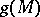into a locally flat submanifold of.

2) Letbe a-dimensional polyhedron. A topological imbedding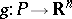is called wild if there does not exist a homeomorphism ofonto itself which would convertinto a polyhedron (i.e. into a body having a certain triangulation) in.

3) Letbe a-dimensional locally compact space. A topological imbedding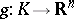is called wild if there does not exist a homeomorphism ofonto itself which would convert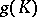into a subset of the-dimensional Menger compactum.

If the dimensionand if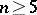, then the properties introduced in all three cases are characterized by the following locally homotopic property: An imbedding is wild if and only if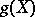does not satisfy the property(cf. Topology of imbeddings). The situation is much more complicated for the codimensions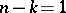and: The problem has been solved for manifolds of codimension 1 for, but has not been fully solved for imbeddings of codimension 2 both for manifolds and for polyhedra. All that has been said is also meaningful ifis an-dimensional manifold — topological or piecewise linear.

How to Cite This Entry:
Wild imbedding. Encyclopedia of Mathematics. URL: http://encyclopediaofmath.org/index.php?title=Wild_imbedding&oldid=18211
This article was adapted from an original article by M.A. Shtan'ko (originator), which appeared in Encyclopedia of Mathematics - ISBN 1402006098. See original article